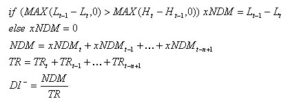## What is a Negative Directional Indicator?

A negative directional indicator (known as negative DI) is a technical measure of a downtrend's momentum.

## How Does a Negative Directional Indicator Work?

Mathematically speaking, a negative directional indicator exists when the difference in a security's low price for two periods is bigger than the difference in the security's high price over the same two periods.

Intuitively, this means a negative directional indicator occurs when:

(Prior Low Price - Current Low Price) > (Current High Price - Prior High Price)

Let's say that all of the stocks in Company XYZ's industry have been declining in value over the last week. John isn’t sure if the trend has enough strength to warrant shorting some Company XYZ stock. To determine the answer, John calculates the negative DI for Company XYZ.

The full formula for calculating Negative DI is:where L = daily low, H = daily high, TR = the True Range for the day, and NDM = negative directional movement.

## Why Does a Negative Directional Indicator Matter?

Most investors can tell whether a security is trending up or down, but they also want to know when a trend is sustainable -- this is why so many technical analysts turn to the DI. Though the math is complex, the idea is that when a negative DI rises above a positive DI, many analysts interpret this as a sign that a new downtrend is beginning, thus making it a sell signal. Likewise, a buy signal occurs when a negative DI falls below a positive DI.

It is important to note, however, that positive and negative DIs intersect frequently. Thus, DI interpretations do not prevent whipsaws. Smart investors will always use other forms of technical and fundamental analysis to confirm what negative DI is suggesting.